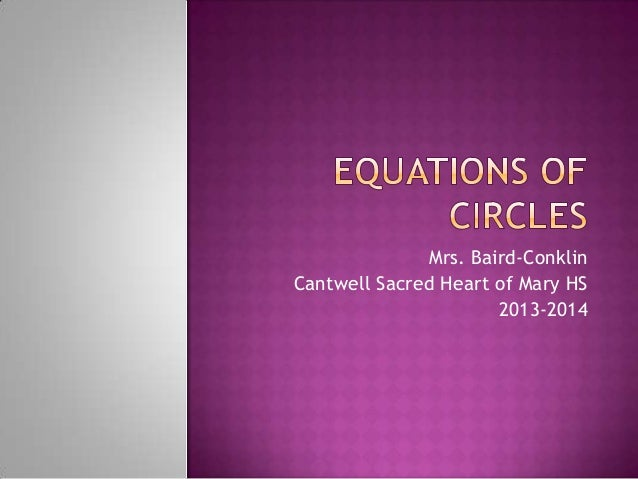Successfully reported this slideshow.Upcoming SlideShare
×

# Equations of circles power point

Circles equations - how to graph a circle from an equation, how to write an equation from a graph.

• Full Name
Comment goes here.

Are you sure you want to Yes No### Equations of circles power point

1. 1. Mrs. Baird-Conklin Cantwell Sacred Heart of Mary HS 2013-2014
2. 2. The center of a circle is given by (h, k) The radius of a circle is given by r The equation of a circle in standard form is (x – h)2 + (y – k)2 = r2 Slide 1
3. 3. Circle A The center is (16, 10) The radius is 10 The equation is (x – 16)2 + (y – 10)2 = 100 Slide2
4. 4. Circle B The center is (4, 20) The radius is 10 The equation is (x – 4)2 + (y – 20)2 = 100 Slide 3
5. 5. Circle O The center is (0, 0) The radius is 12 The equation is x 2 + y 2 = 144 Slide 4
6. 6. (x – 3)2 + (y – 2)2 = 9 Center (3, 2) Radius of 3 Slide 5
7. 7. (x + 4)2 + (y – 1)2 = 25 Center (-4, 1) Radius of 5 Slide 6
8. 8. (x – 5)2 + y2 = 36 Center (5, 0) Radius of 6 Slide 7
9. 9. Write the standard equation of the circle: Center (4, 7) Radius of 5 (x – 4)2 + (y – 7)2 = 25 Slide 8
10. 10. Write the standard equation of the circle: Center (-3, 8) Radius of 6.2 (x + 3)2 + (y – 8)2 = 38.44 Slide 9
11. 11. Write the standard equation of the circle: Center (2, -9) Radius of (x – 2)2 + (y + 9)2 = 11 11 Slide 10
12. 12. Write the standard equation of the circle: Center (0, 6) Radius of x 2 + (y – 6)2 = 7 7 Slide 11
13. 13. Write the standard equation of the circle: Center (-1.9, 8.7) Radius of 3 (x + 1.9)2 + (y – 8.7)2 = 9 Slide 12